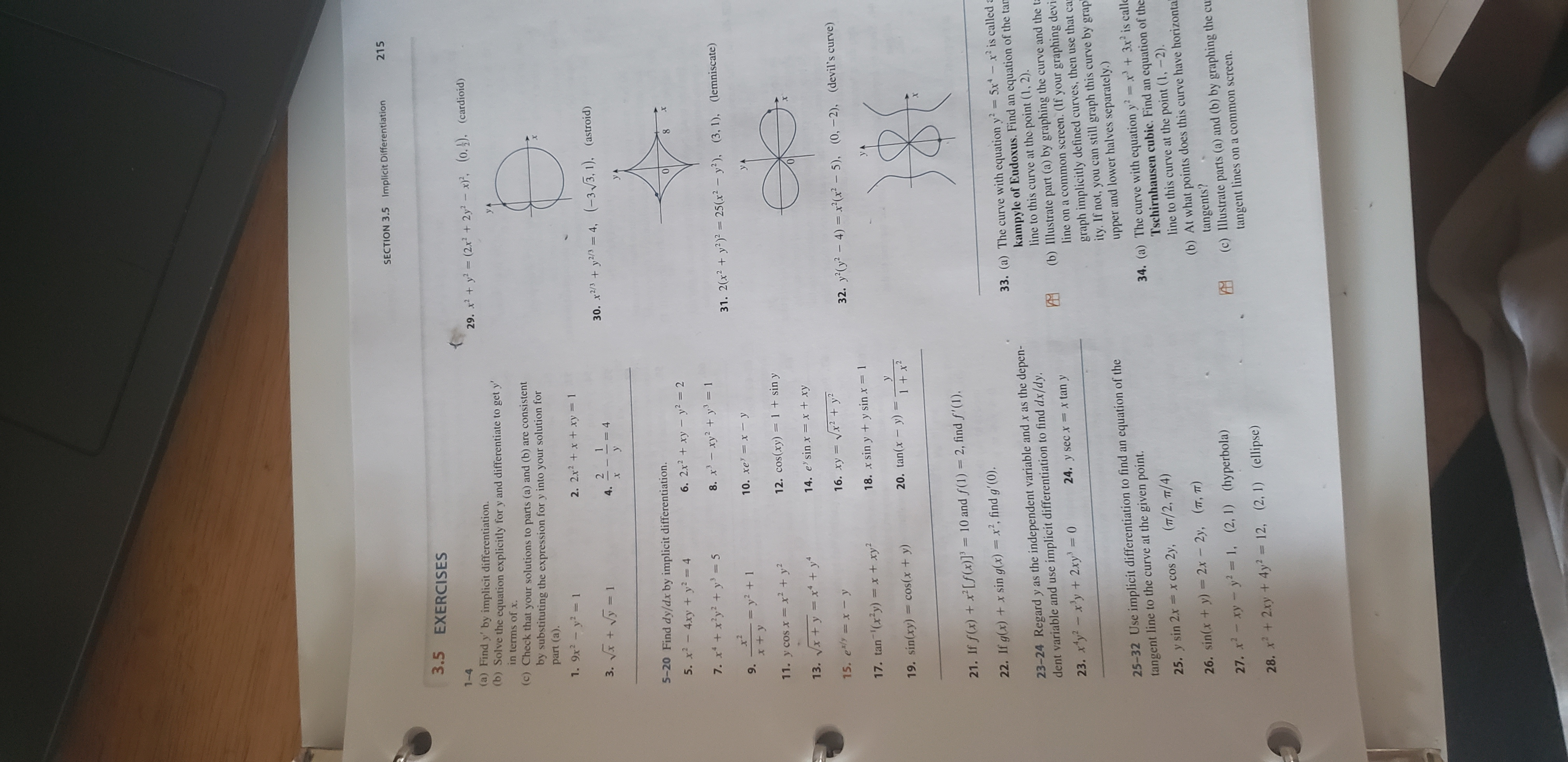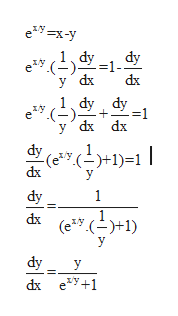# SECTION 3.5 Implicit Differentiation2153.5 EXERCISES1-429. xy (2x 2y2 x, (0.). (cardioid)(a) Find y' by implicit differentiation.(b) Solve the equation explicitly for y and differentiate to get yin terms of x.(c) Check that your solutions to parts (a) and (b) are consistentby substituting the expression for y into your solution forpart (a).1. 9x2 y2 12. 2x2x + xy 13. 124.30. x2/y2/4, (-3/3, 1), (astroid)- =45-20 Find dy/dx by implicit differentiation.5. x2-4xy + y2 46. 2x2+xy y2 = 27. x+x'y2y 58. x-xy2 y= 131. 2(x2y225(x2- y2), ( 3, 1), (lemniscate)x210. xe xy9.= y2 + 1x+ y12. Cos(xy) 1 + sin y11. y cos x x2 + y14. e' sin x x + xy13. Vx +y x+ yVx2 +y15. e xy16. xy32. y(y 4) x(x -5), (0,-2), (devil's curve)17. tan (xy) x + xy18. x sin y + y sin x 120. tan(x y)19. sin(xy) cos(x + y)1x221. If f(x) +x[f (x)]' = 10 and f(1) = 2, find f'(1).22. If g(x) +x sin g(x) x, find g' (0).33. (a) The curve with equationy 5x-x2 is called akampyle of Eudoxus. Find an equation of the tamline to this curve at the point (1, 2).(b) Illustrate part (a) by graphing the curve and the tline on a common screen. (If your graphing devi-graph implicitly defined curves, then use that caity. If not, you can still graph this curve by grapupper and lower halves separately.)23-24 Regard y as the independent variable and x as the depen-dent variable and use implicit differentiation to find dx/dy.24. y sec x x tan y23. xy2-x'y + 2xy 025-32 Use implicit differentiation to find an equation of thetangent line to the curve at the given point.(T/2, T/4)x +3x2 is calle34. (a) The curve with equation yTschirnhausen cubic. Find an equation of theline to this curve at the point (1, -2).(b) At what points does this curve have horizontatangents?(c) Illustrate parts (a) and (b) by graphing the cutangent lines on a common screen.25. y sin 2x x cos 2y,(T, T)2x 2y,26. sin(x + y)27. xxy y2= 1, (2, 1) (hyperbola)28. x2xy +4y 12, (2, 1) (ellipse)

Question

I need help with question 15 in Section 3.5, page 215, of the James Stewart Calculus Eighth Edition textbook.help_outlineImage TranscriptioncloseSECTION 3.5 Implicit Differentiation 215 3.5 EXERCISES 1-4 29. xy (2x 2y2 x, (0.). (cardioid) (a) Find y' by implicit differentiation. (b) Solve the equation explicitly for y and differentiate to get y in terms of x. (c) Check that your solutions to parts (a) and (b) are consistent by substituting the expression for y into your solution for part (a). 1. 9x2 y2 1 2. 2x2x + xy 1 3. 1 2 4. 30. x2/y2/4, (-3/3, 1), (astroid) - =4 5-20 Find dy/dx by implicit differentiation. 5. x2-4xy + y2 4 6. 2x2+xy y2 = 2 7. x+x'y2y 5 8. x-xy2 y= 1 31. 2(x2y225(x2- y2), ( 3, 1), (lemniscate) x2 10. xe xy 9. = y2 + 1 x+ y 12. Cos(xy) 1 + sin y 11. y cos x x2 + y 14. e' sin x x + xy 13. Vx +y x+ y Vx2 +y 15. e xy 16. xy 32. y(y 4) x(x -5), (0,-2), (devil's curve) 17. tan (xy) x + xy 18. x sin y + y sin x 1 20. tan(x y) 19. sin(xy) cos(x + y) 1x2 21. If f(x) +x[f (x)]' = 10 and f(1) = 2, find f'(1). 22. If g(x) +x sin g(x) x, find g' (0). 33. (a) The curve with equationy 5x-x2 is called a kampyle of Eudoxus. Find an equation of the tam line to this curve at the point (1, 2). (b) Illustrate part (a) by graphing the curve and the t line on a common screen. (If your graphing devi- graph implicitly defined curves, then use that ca ity. If not, you can still graph this curve by grap upper and lower halves separately.) 23-24 Regard y as the independent variable and x as the depen- dent variable and use implicit differentiation to find dx/dy. 24. y sec x x tan y 23. xy2-x'y + 2xy 0 25-32 Use implicit differentiation to find an equation of the tangent line to the curve at the given point. (T/2, T/4) x +3x2 is calle 34. (a) The curve with equation y Tschirnhausen cubic. Find an equation of the line to this curve at the point (1, -2). (b) At what points does this curve have horizonta tangents? (c) Illustrate parts (a) and (b) by graphing the cu tangent lines on a common screen. 25. y sin 2x x cos 2y, (T, T) 2x 2y, 26. sin(x + y) 27. xxy y2= 1, (2, 1) (hyperbola) 28. x2xy +4y 12, (2, 1) (ellipse) fullscreen
check_circleExpert Solution

in parametric form just consider the dependent and independent variable. also c...help_outlineImage Transcriptionclosee*у-х-у у dx dx dy dy - 1 у dx dx е* (-). ефн! dy "-)+1)=1 | dx dy 1 dx (e*У. (—)+1) dy у dx e*у+1 fullscreen

### Want to see the full answer?

See Solution

#### Want to see this answer and more?

Solutions are written by subject experts who are available 24/7. Questions are typically answered within 1 hour*

See Solution
*Response times may vary by subject and question
Tagged in

### Integration